# Adjustable Curve Blend - setting G3 leads to only G2 Continuity

Hello, first Post.

I have two lines like in this image, and I want to do an adjustable curve blend operation on them.

In the Adjustable Curve Blend dialogue I specify both ends to be G3 continous.

Next, I use the resulting curve to split the vertical line.

Now, if I check the resulting lower half of the vertical line and the blend curve for continuity, they are G2.

I had expected G3 continuity here, because I had specified G3 in the Adjustable Curve Blend dialogue.
Can somebody explain to me, why its G2, and, can anybody tell me what I can do to get this G3?

Thank you very much,

Frido

though the following below is what the help reports, it seems that is still a bug, not sure if it should not just also evaluate above g2… otherwise it should just also point out that continuities above g2 will not be shown due to bla bla like @pascal tried to explain.

# GCon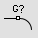Analyze|Analyze

Curve >

Geometric Continuity|

The GCon command reports the difference of position, radius, tangency and curvature between two curve ends.

#### Steps

1. Select the first curve near the end that coincides with the end of another curve.
2. Select the second curve near the coinciding end.
Tangency and curvature continuity information display on the command line.

## Continuity

Position (G0) Position (G0 continuity) measures location only. If the end points of each curve are in the same location in space, the curves are position continuous (G0) at the ends. In other words, the two curves in question touch each other at their end points.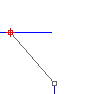Tangency (G1) Tangency (G1 continuity) measures position and curve direction at the ends. in other words, the two curves not only touch, but they go the same direction at the point where they touch.

The direction is determined by the first and second point on each curve. If these two points fall on a line, the two curves are tangent at the ends.

The first derivative of the two curves is equal at the point where they touch.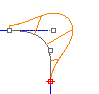Curvature (G2) Curvature continuity (G2 continuity) between two curves measures position, direction, and radius of curvature at the ends. If the radius of curvature is the same at the common end point, curves are curvature continuous (G2). In other words, the curves not only go the same direction when they meet, but also have the same radius at that point. This condition is not easy to determine by just looking at where the points are located.

Both the first and second derivatives of the equations are equal at that point.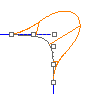G3 G3 continuity adds a third requirement: planar acceleration. Curves that are G3 continuous touch, go the same direction, have the same radius, and that radius is accelerating at the same rate at a certain point.

G3 continuous curves have equal third derivatives.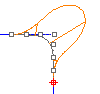G4 G4 continuity is very seldom used, but can be important in certain isolated cases. G4 continuous curves have all the same requirements as G3 curves, but their curvature acceleration is equal in three dimensions.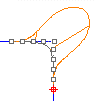Hello, and thank you very much for your quick response.
I did the curvature graph, and I would say that looks good. The curvature smoothly goes down to zero at the transition to the straight line, which makes sense.

I realized, that Gcon lists the radius of curvature value as 0.00… . If I got that right, that already means it is G3. So it evaluates correctly, but then calls it G2.

cheers and best regards.

Frido

G3 means the rate of change of curvature with distance along the curve is continuous, along with curvature, tangent direction and position. Two curves can be G3 without the curvature being 0.

For the particular point in this example one of the curves is straight which means the curvature is 0 and the rate of change of curvature is also 0. The curvature graph appears to show the rate of change of curvature to be 0.

1 Like

Hello,

thank you for the clarification.
Given, that the curvature is approching 0 smoothly and reaches 0 at the point where the two curves meet, I consider them G3 and move on with them.
In doing so, I already have a new question, which I will try to approach in a new post.

Thank you very much for your help,

Frido